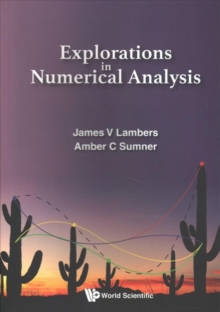# Explorations In Numerical Analysis Paperback / softback

#### Description

This field pertains to the design, analysis, and implementation of algorithms for the approximate solution of mathematical problems that arise in applications spanning science and engineering, and are not practical to solve using analytical techniques such as those taught in courses in calculus, linear algebra or differential equations.

Topics covered include error analysis, computer arithmetic, solution of systems of linear equations, least squares problems, eigenvalue problems, polynomial interpolation and approximation, numerical differentiation and integration, nonlinear equations, optimization, ordinary differential equations, and partial differential equations.

For each problem considered, the presentation includes the derivation of solution techniques, analysis of their efficiency, accuracy and robustness, and details of their implementation, illustrated through the MATLAB programming language.

This text is suitable for a year-long sequence in numerical analysis, and can also be used for a one-semester course in numerical linear algebra.

#### Information

• Format: Paperback / softback
• Pages: 676 pages
• Publisher: World Scientific Publishing Co Pte Ltd
• Publication Date:
• Category: Numerical analysis
• ISBN: 9789813209978

£56.00

£40.22

Free delivery within the UK